# Trigonometry Question Help

## Homework Statement

The machine tool diagram on the right shows a symmetric worm
thread, in which a circular roller of diameter 1.5 inches sits.
Find the amount d that the top of the roller rises above the
top of the thread, given the information in the diagram. (Hint:
Extend the slanted sides of the thread until they meet at a point.)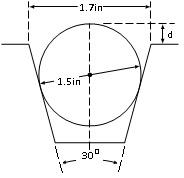## The Attempt at a Solution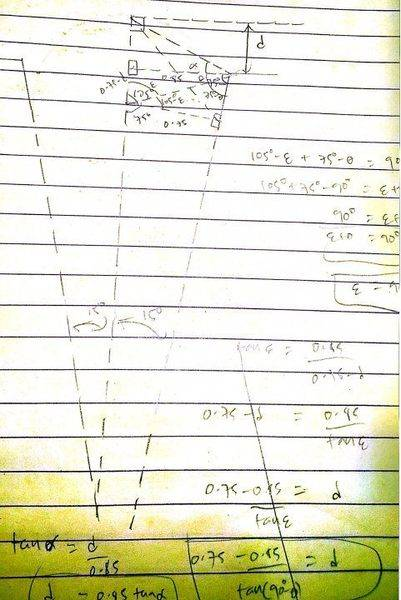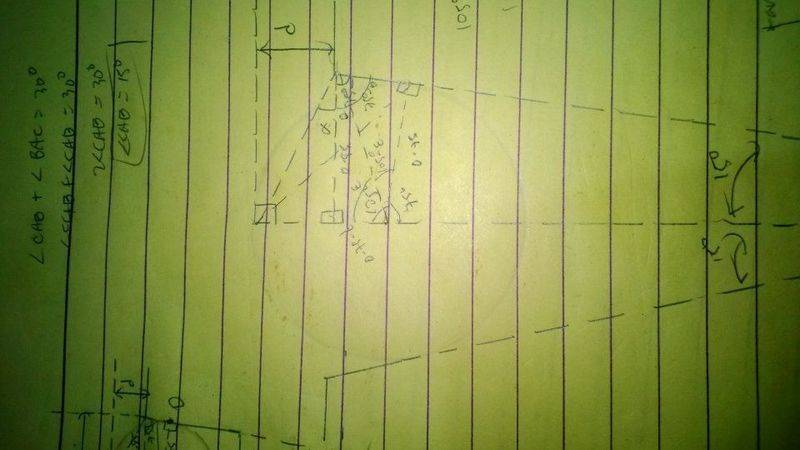LCKurtz
Homework Helper
Gold Member
Unreadable and sizeways images? You have to be kidding. My suggestion would be to post a single readable image with the picture and labeled variables, such as ##\alpha##, ##d##, ##r##, and possibly others. Then write (type!) your solution in terms of known variables. So you get ##d = \text{ some formula}##. Only put in the values at the end. Then your solution would be readable and understandable.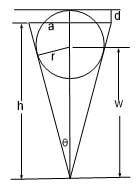Now, you know ##r,~a,~\theta##. Solve for ##d## in terms of them.

Last edited:
So we have r = 0.75, h = 3.1722 and w = 2.7990. In the end it will be r - d = h - w which would be d = 0.3768. I looked at the back of the book the answer is 0.476. And the reason is because r does not make a right triangle with w as its leg. There is a slight space with the circle and the symmetric thread. Look at the picture below: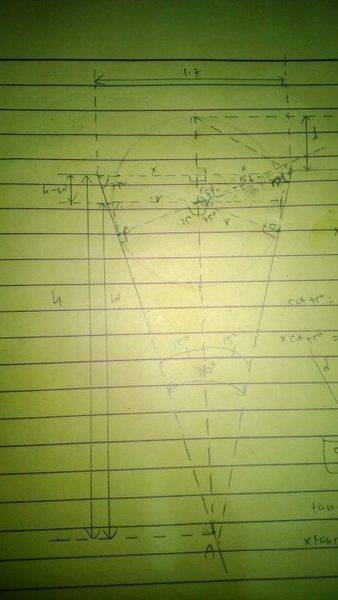LCKurtz
Homework Helper
Gold Member
Unreadable and sizeways images? You have to be kidding. My suggestion would be to post a single readable image with the picture and labeled variables, such as ##\alpha##, ##d##, ##r##, and possibly others. Then write (type!) your solution in terms of known variables. So you get ##d = \text{ some formula}##. Only put in the values at the end. Then your solution would be readable and understandable.
View attachment 114142
Now, you know ##r,~a,~\theta##. Solve for ##d## in terms of them.

So we have r = 0.75, h = 3.1722 and w = 2.7990. In the end it will be r - d = h - w which would be d = 0.3768. I looked at the back of the book the answer is 0.476. And the reason is because r does not make a right triangle with w as its leg. There is a slight space with the circle and the symmetric thread.

Again, your image is impossible to read. And, yes, ##r## is perpendicular to its leg. You haven't shown readable equations (type them in here, don't post images) for ##h## and ##w## so I don't know what you are doing wrong. It doesn't help when you just post numbers. Using my figure, you should get ##w = 2.897## and ##h = 3.172##. This will get you ##d=.4755##.
[Edit, added:] I think I see where you may be going wrong. Notice that ##w## is the hypotenuse of the right triangle and use that.

Last edited:
Mark44
Mentor
Epsilon, I have locked this thread. Please start a new thread with an image that can be read, and with equations typed in as text, as LCKurtz asks. It's possible to use features available at this site to enter each equation you show. Look under INFO in the menu bar across the top of the screen, in the Help/How-to submenu. There's a tutorial on LaTeX (https://www.physicsforums.com/help/latexhelp/), that can be used to write just about any mathematical equation.

Also, please label the points in your drawing for ease of reference.

Last edited: